# NumbersPage 1

#### WATCH ALL SLIDES

Slide 1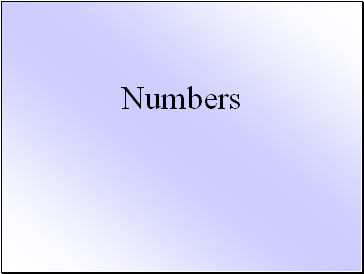Numbers

Slide 2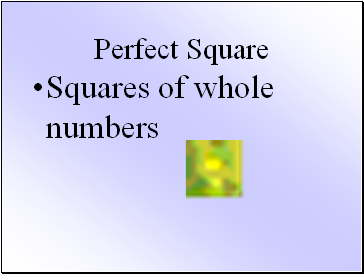## Perfect Square

Squares of whole numbers

Slide 3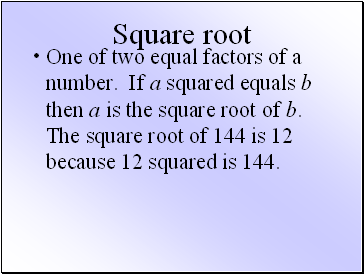## Square root

One of two equal factors of a number. If a squared equals b then a is the square root of b. The square root of 144 is 12 because 12 squared is 144.

Slide 4The sign used to represent a nonnegative square root.

Slide 5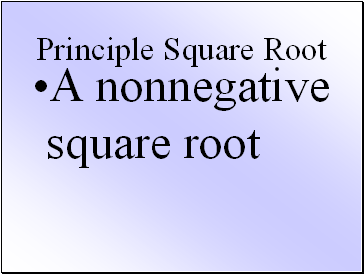## Principle Square Root

A nonnegative square root

Slide 6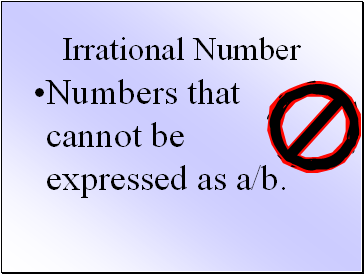## Irrational Number

Numbers that cannot be expressed as a/b.

Slide 7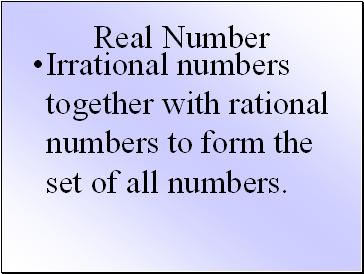## Real Number

Irrational numbers together with rational numbers to form the set of all numbers.

Slide 8## Natural Numbers

1, 2, 3, 4 .

Slide 9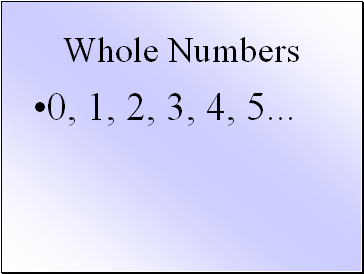## Whole Numbers

0, 1, 2, 3, 4, 5 .

Slide 10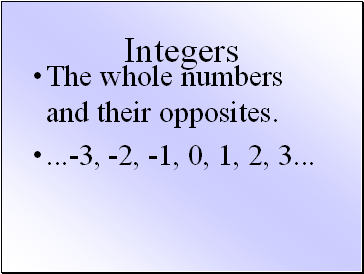## Integers

The whole numbers and their opposites.

.-3, -2, -1, 0, 1, 2, 3 .

Slide 11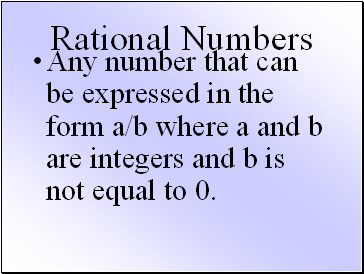## Rational Numbers

Any number that can be expressed in the form a/b where a and b are integers and b is not equal to 0.